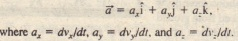# REVIEW AND SUMMARY MOTION IN TWO THREE DIMENSIONS

REVIEW  AND SUMMARY MOTION IN TWO THREE DIMENSIONS

Position Vector The location of a particle to the origin of a coordinate system is given by a position vector ,which in unit-vector notation isHere  the vector components of position vector F, and x, y, and z are its scalar components (as well as the coordinates of the particle), A position vector is described by a magnitude  and one or two angles for orientation, or by its vector or scalar components.

Displacement if  a particle moves so that its position vector changes from r) to  then the particle’s displacementAverage Acceleration and (Instantaneous) Acceleration

If a particle’s velocity changes from  in time interval  its average acceleration duringIn unit-vector notation,Projectile Motion Projectile motion is the motion of a particle that is launched with an initial velocity. During its flight, the particle’s horizontal acceleration is zero and its vertical acceleration is the free-fall acceleration upward is taken to be a positive.Uniform Circular Motion, If a particle travels along a circle or circular arc with radius I’ at constant speed v, it is in uniform circular motion and has an acceleration 0 of magnitude.Relative Motion When two frames of reference A and B are moving relative to each other at constant velocity, the velocity of a particle P as measured by an observer in frame A usually differs  from that measured from frame B.